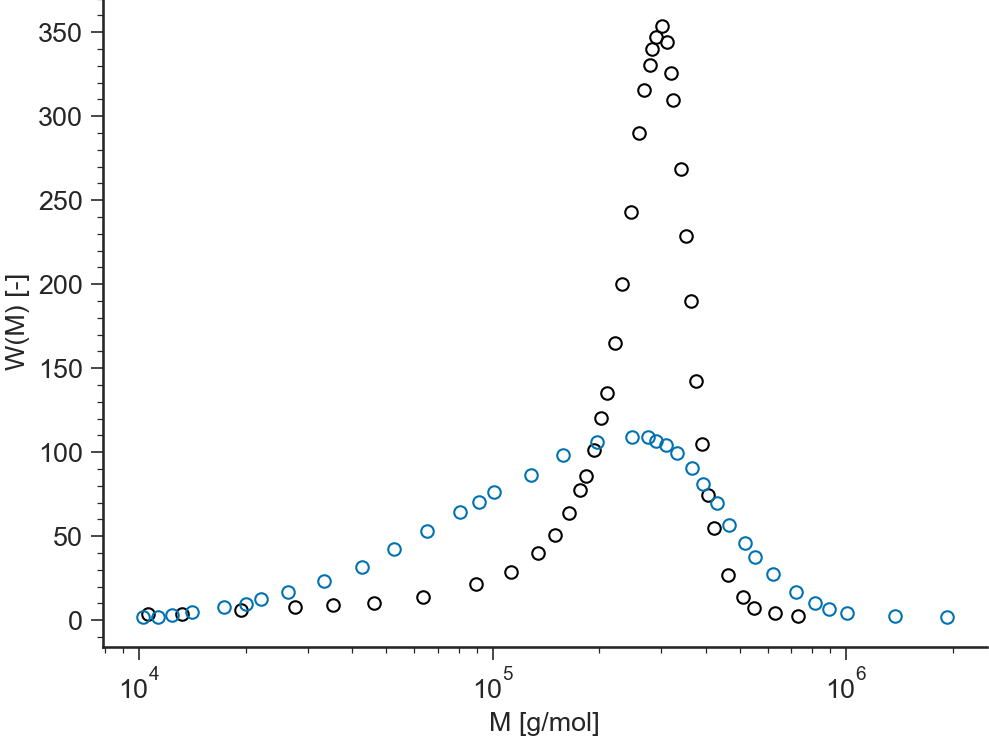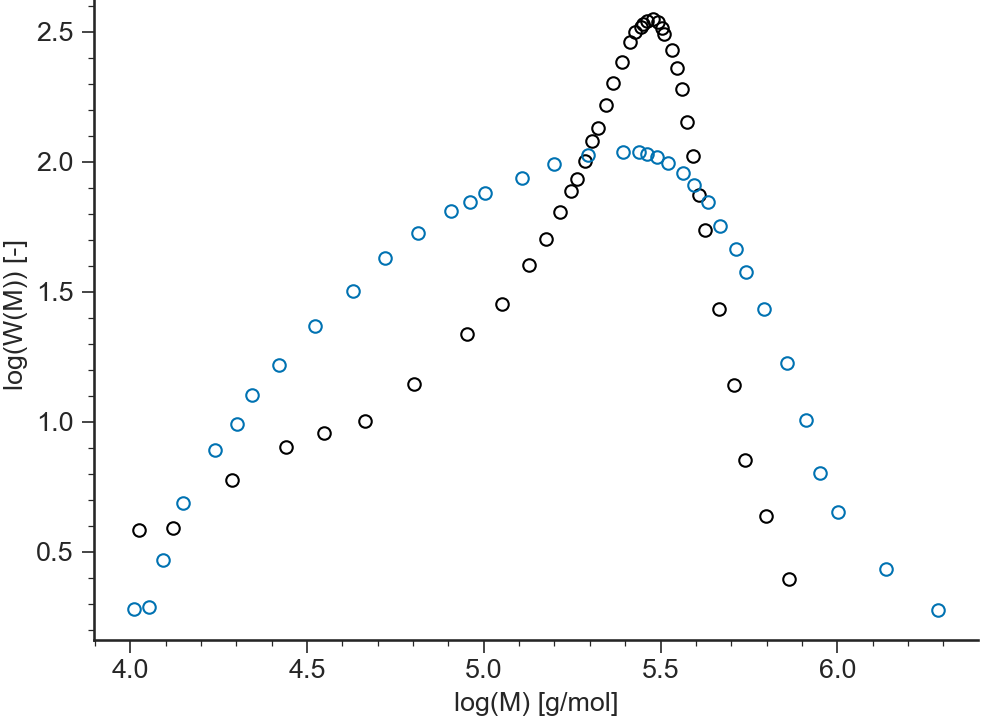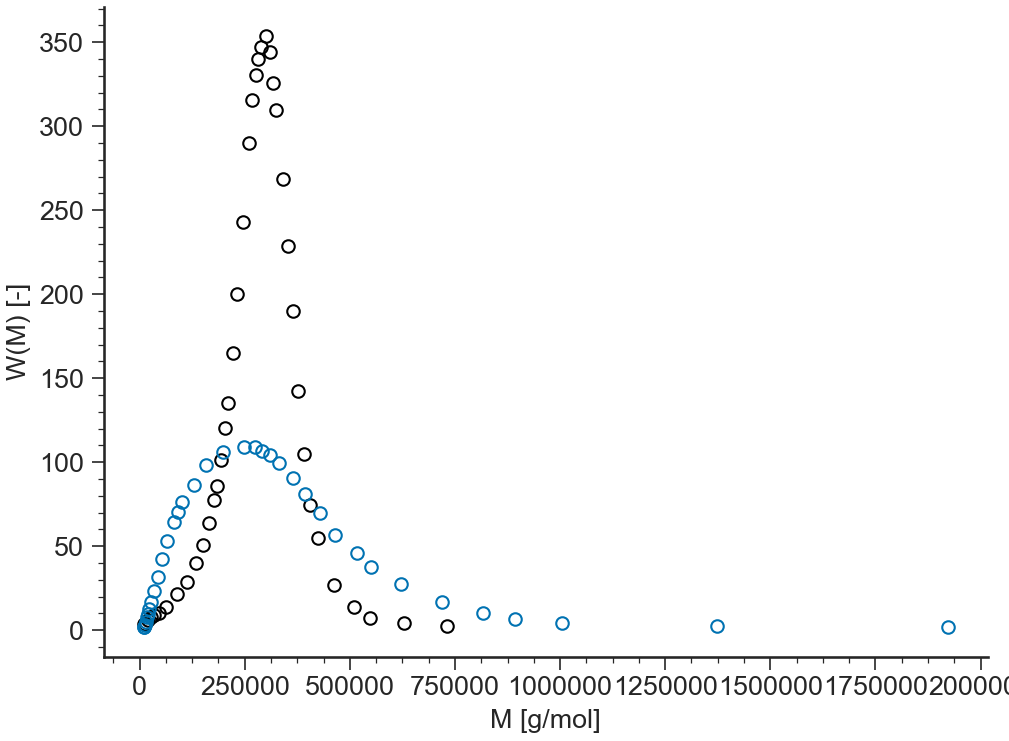# MWD: General description¶

## Purpose¶

Application to analyze Molecular Weight Distributions

## Data Files¶

• The first line of the file should contain the sample parameters separated by semi-colons (;). It may contain any number of parameters which will be read and saved as file-parameter in RepTate.

• Then the data columns should appear, separated by spaces or tabs.

### .gpc extension¶

Text files with .gpc extension should be organised as follows:

• .gpc files should provide parameter values for

1. number-average molar mass, $$M_n$$

2. weight-average molar mass, $$M_w$$

3. polydispersity index, $$\text{PDI}$$

• 2 columns separated by spaces or tabs containing respectively:

1. molar mass, $$M$$,

2. weight associated, $$\dfrac{\text d w(\log M)}{\text d \log M}$$.

A correct .gpc file looks like:

Mn=100;Mw=250;PDI=2.5
0.1148E+03 0.5000E-03
0.1514E+03 0.1500E-02
0.1995E+03 0.1667E-02
0.2630E+03 0.1750E-02
0.3467E+03 0.5250E-02
0.4571E+03 0.6750E-02
...        ...


### .reac extension¶

Text files with .reac extension should be organised as follows:

• .reac files are not expected to provide any specific parameter values.

• 4 columns separated by spaces or tabs containing respectively:

1. molar mass, $$M$$,

2. weight associated, $$\dfrac{\text d w(\log M)}{\text d \log M}$$,

3. the g-factor, $$g(M)$$,

4. number of branch per 1000 carbon, $$\log_{10}(g(M))$$.

A correct .reac file looks like:

0.1148E+03 0.5000E-03 0.1000E+01 0.0000E+00
0.1514E+03 0.1500E-02 0.1000E+01 0.0000E+00
0.1995E+03 0.1667E-02 0.1000E+01 0.0000E+00
0.2630E+03 0.1750E-02 0.1000E+01 0.0000E+00
0.3467E+03 0.5250E-02 0.1000E+01 0.0000E+00
0.4571E+03 0.6750E-02 0.9977E+00 0.3765E+00
...        ...        ...        ...


## Views¶

### log-lin¶

BaseApplicationMWD.view_WM()[source]

$$W(M)$$ vs $$M$$

(Molecular weight in logarithmic scale)### log-log¶

BaseApplicationMWD.view_logWM()[source]

$$\log(W(M))$$ vs $$\log(M)$$### lin-lin¶

BaseApplicationMWD.view_WM()[source]

$$W(M)$$ vs $$M$$